In the verge of coronavirus pandemic, we are providing FREE access to our entire Online Curriculum to ensure Learning Doesn't STOP!

# Two-Point Form

Go back to  'Coordinate-Geometry'

Two points lying on a line will also uniquely determine the line. The following figure shows two points $$A\left( {{x_1},{y_1}} \right)$$ and $$B\left( {{x_2},{y_2}} \right)$$ lying on a line: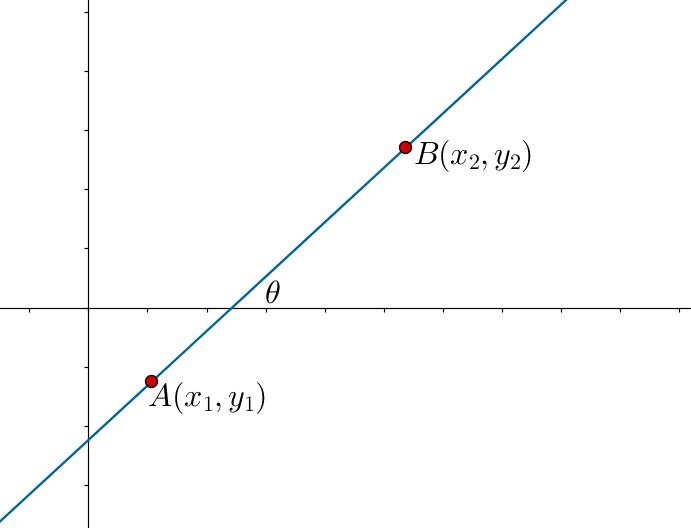How do we find the equation of this line? Easy – we first find the slope of this line using the two points, and then use the point-slope form.

Observe the following figure, which shows that $$\angle BAC = \theta$$ (the inclination angle of the line):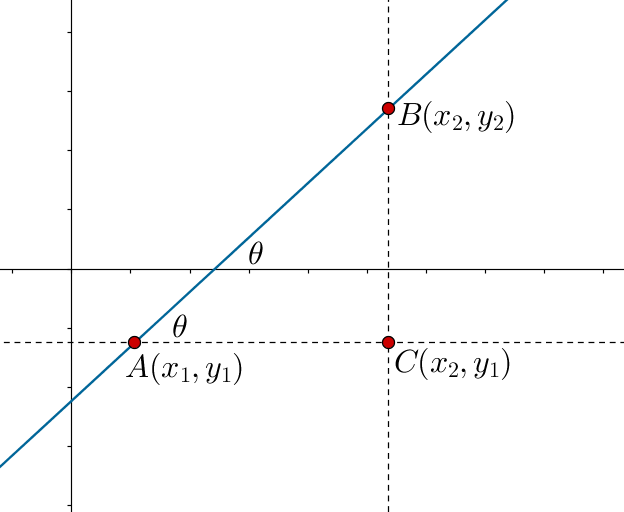Clearly, the slope of the line is

$m = \frac{{BC}}{{AC}} = \frac{{{y_2} - {y_1}}}{{{x_2} - {x_1}}}$

Thus, using the point-slope form, the equation of the line is

\begin{align}&y - {y_1} = m\left( {x - {x_1}} \right)\\&\Rightarrow \,\,\,y - {y_1} = \left( {\frac{{{y_2} - {y_1}}}{{{x_2} - {x_1}}}} \right)\left( {x - {x_1}} \right)\\&\Rightarrow \,\,\,\frac{{y - {y_1}}}{{x - {x_1}}} = \frac{{{y_2} - {y_1}}}{{{x_2} - {x_1}}}\end{align}

This is known as the two-point form of a line’s equation, as we have used two points lying on the line to specify it.

Example 1: Find the equation of the line passing through $$A\left( {1,2} \right)$$ and $$B\left( { - 1,3} \right)$$ .

Solution: The following figure depicts this line: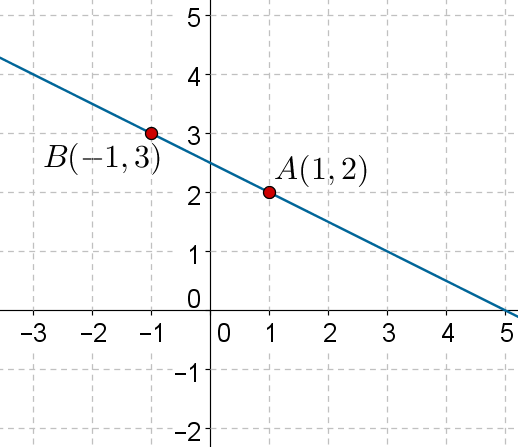Using the two-point form, we have:

\begin{align}&\frac{{y - {y_1}}}{{x - {x_1}}} = \frac{{{y_2} - {y_1}}}{{{x_2} - {x_1}}}\\&\Rightarrow \,\,\,\frac{{y - 2}}{{x - 1}} = \frac{{3 - 2}}{{ - 1 - 1}}\\&\Rightarrow \,\,\,\frac{{y - 2}}{{x - 1}} = \frac{1}{{ - 2}}\\&\Rightarrow \,\,\, - 2y + 4 = x - 1\\&\Rightarrow \,\,\,x + 2y - 5 = 0\end{align}

Example 2: Find the y-intercept of the line passing through the points $$A\left( {3, - 2} \right)$$ and $$B\left( { - 1,3} \right)$$ .

Solution: The following figure shows the specified line: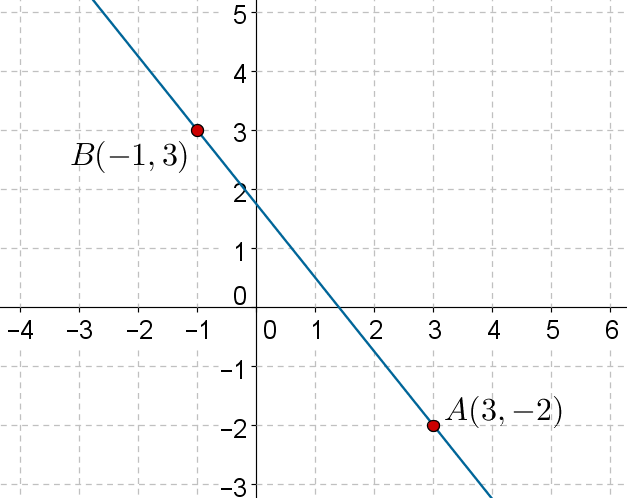Using the two-point form, the equation of the line is:

\begin{align}&\frac{{y - {y_1}}}{{x - {x_1}}} = \frac{{{y_2} - {y_1}}}{{{x_2} - {x_1}}}\\&\Rightarrow \,\,\,\frac{{y - \left( { - 2} \right)}}{{x - 3}} = \frac{{3 - \left( { - 2} \right)}}{{ - 1 - 3}}\\&\Rightarrow \,\,\,\frac{{y + 2}}{{x - 3}} = \frac{5}{{ - 4}}\\&\Rightarrow \,\,\, - 4y - 8 = 5x - 15\\&\Rightarrow \,\,\,5x + 4y - 7 = 0\end{align}

Now, we rearrange this equation into the slope-intercept form:

\begin{align}&4y = - 5x + 7\\&\Rightarrow \,\,\,y = \left( { - \frac{5}{4}} \right)x + \frac{7}{4}\end{align}

It is evident that the y-intercept is

$c = \frac{7}{4}$

Example 3: Write the equation of the line whose x-intercept is a and y-intercept is b, as shown in the following figure: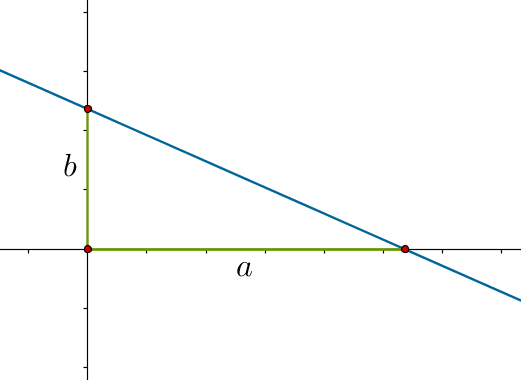Solution: The line passes through the points $$A\left( {a,0} \right)$$ and $$B\left( {0,b} \right)$$. Using the two-point form, the equation of the line is

\begin{align}&\frac{{y - {y_1}}}{{x - {x_1}}} = \frac{{{y_2} - {y_1}}}{{{x_2} - {x_1}}}\,\,\, \Rightarrow \,\,\,\frac{{y - 0}}{{x - a}} = \frac{{b - 0}}{{0 - a}}\\&\Rightarrow \,\,\,\frac{y}{{x - a}} = \frac{b}{{ - a}}\,\,\, \Rightarrow \,\,\, - ay = bx - ab\\&\Rightarrow \,\,\,bx + ay = ab\,\,\, \Rightarrow \,\,\,\frac{x}{a} + \frac{y}{b} = 1\end{align}

This form of a line’s equation is known as the intercept form – when the intercepts of the lines on the two axes are known, we can use this equation directly. For example, consider a line whose intercepts on the x and y axes are 3 and $$- 5$$ respectively: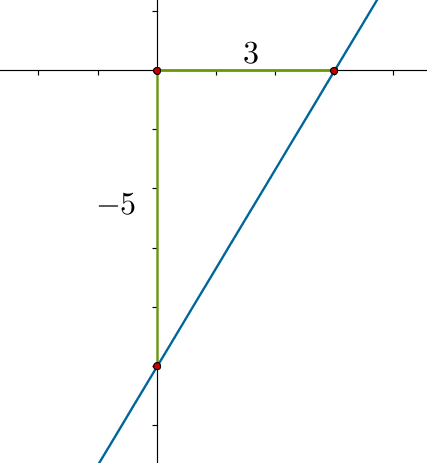The equation of this line, using the intercept form, will be

\begin{align}&\frac{x}{3} + \frac{y}{{ - 5}} = 1\\&\Rightarrow \,\,\, - 5x + 3y = - 15\\&\Rightarrow \,\,\,5x - 3y - 15 = 0\end{align}

Coordinate Geometry
Grade 9 | Questions Set 1
Coordinate Geometry
Coordinate Geometry
Grade 10 | Questions Set 1
Coordinate Geometry